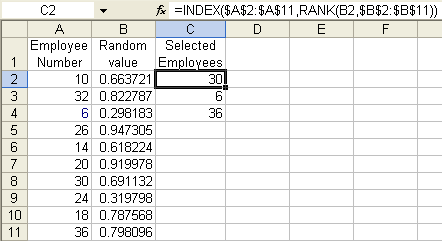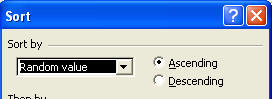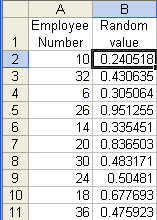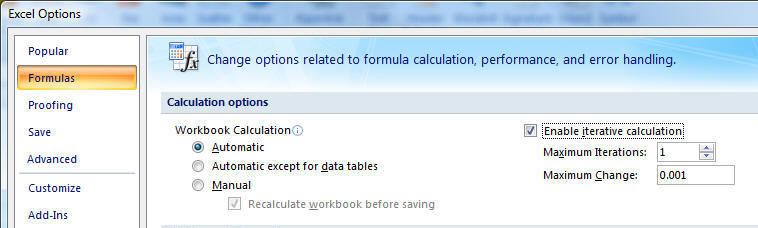﻿ Random Selection
You are on the Home/Excel/Tutorials/Random Selection pageShare Your

# Select elements at random without repetition

A common requirement is the selection of some number of elements at random and without repetition from a larger group.  This could be to select raffle winners or door prize winners.  It could also be to select random employees (or athletes) for a drug test.  It could be to randomize the list of questions in a test.  Of course, there are other applications of this technique.

The example used to illustrate each of the methods below is the selection of 3 employees from a group of 10.  Of course, this example is easily extended to select m entries from a list of n elements, where m <= n.

There are several different ways to select elements at random without repetition in Excel.  Some of the techniques generate a static list in the sense that the list changes only when the user explicitly requests a change.  Other techniques yield a list that changes each time Excel recalculates the worksheet.  And, there is one technique that some believe to generate static random numbers but as we will see it is only pseudo-static.

## Generate a random list (with an intermediate random number list) that changes with each recalculation

There may be instances where we want the list to change each time Excel recalculates the worksheet.  In addition to applications such as running a simulation, these techniques also serve as building blocks for those used to generate static random numbers.  One of the techniques shown below is a worksheet-only approach and the others are all VBA-based.

Generate a recalculating random list using the RAND() and RANK() functions

VBA functions to generate a recalculating random list

## Generate a static random selection (with static intermediate random numbers)

On many occasions it is important to generate a static random selection, where a static random number is one that changes only when the user wants the change to occur.  This could be because all thetasks associated with the list must be completed first.  An example would be carrying out some sort of test for each of the selected elements.  It could also be that we never want the list to change as in the case of selecting lottery winners (or raffle winners or winners of door prizes).  Another possibility is that we want to keep the list static until we complete some sort of audit based on the selected items, as in the case of a financial or accounting audit.

Use the RAND() function and sort the result

Use iterative calculations to generate a static list

Generate a random selection using a VBA subroutine

A pseudo-static random list with a User Defined Function (UDF)

Select a random subset without repetition -- use the Rank function

The advantage of this method is that the process of regenerating a new list is as easy as recalculating the worksheet (on a Windows machine, pressing the F9 key).  The disadvantage is that as soon as a recalculation is done, the previous result is lost forever -- unless, of course, it was saved somewhere else.

Suppose the group from which the selection has to be made is in A1:A11.

Then, in the adjacent column, enter the formula =RAND().  In the next column over, enter the formula =INDEX(\$A\$2:\$A\$11,RANK(B2,\$B\$2:\$B\$11)).   Copy C2 down to cover as many cells as desired selections.Select a random subset without repetition -- use a user defined function (UDF)

There are three procedures described below.  Each is a variant of an efficient single-pass algorithm for generating a random selection from a specified list.

A worksheet range contains the list from which the random selections are made

Return a specified number of random numbers from a set of numbers with a specified lower and upper values

Return a specified number of random values from an user specified array

A worksheet range contains the list from which the random selections are made.

The code below goes into a normal module.  The function RandomSelection is then available for use in a spreadsheet.  Typically, the function should be used as an array-formula.  The function takes a single argument, a worksheet range from the random selections are to be made.  How many selections depends on the number of cells selected for array-entering the function.``` Option Explicit Function RandomSelection(aRng As Range)     Dim myTarg As Range, _         SrcList, Rslt(), _         i As Long, j As Long, k As Long     Application.Volatile     SrcList = aRng.Value     Set myTarg = Application.Caller     With myTarg     If .Areas.Count > 1 Then          RandomSelection = _              "Function can be used only in a single contiguous range"         Exit Function   '<<<<<         End If     If .Rows.Count > 1 And .Columns.Count > 1 Then         RandomSelection = _             "Selected cells must be in a single row or column"         Exit Function   '<<<<<         End If     If .Cells.Count > aRng.Cells.Count Then         RandomSelection = _             "Range specified as argument must contain more cells than output selection"         Exit Function   '<<<<<         End If     ReDim Rslt(1 To IIf(.Rows.Count > 1, .Rows.Count, .Columns.Count))         End With     j = UBound(SrcList, 1)     For i = LBound(Rslt) To UBound(Rslt)         k = Int(Rnd() * (j - LBound(SrcList, 1) + 1)) + LBound(SrcList, 1)         Rslt(i) = SrcList(k, 1)         SrcList(k, 1) = SrcList(j, 1)         j = j - 1         Next i     If myTarg.Rows.Count > 1 Then         RandomSelection = Application.WorksheetFunction.Transpose(Rslt)     Else         RandomSelection = Rslt         End If     End Function```

This is a variant of a single pass algorithm that generates Amount random numbers from a list of numbers with the lowest number specified by Bottom and the highest number specified by TopWhile this can be used as a UDF, the more likely use will be to call it from another VBA procedure.  As a UDF, it would be used as in =TMOptRands(11, 20,5), which returns 5 random numbers from the set (11, 12,...,19, 20).

```Public Function TMOptRands(Bottom As Long, Top As Long, _          Amount As Long) As Variant     Dim i As Long, r As Long, temp As Long         ReDim iArr(Bottom To Top) As Long     For i = Bottom To Top: iArr(i) = i: Next i     For i = 1 To Amount         r = Int(Rnd() * (Top - Bottom + 1 - (i - 1))) _             + (Bottom + (i - 1))         temp = iArr(r): iArr(r) = iArr(Bottom + i - 1): _             iArr(Bottom + i - 1) = temp         Next i     ReDim Preserve iArr(Bottom To Bottom + Amount - 1)     TMOptRands = iArr     End Function```

This is a variant of a single pass algorithm that returns N random elements from the contents of Arr.  The same array is used to return the numbers and can be reused to create another list.  The N random numbers are returned in the lower N array elements.

```Sub Swap(ByRef Arr() As Variant, ByVal i As Long, ByVal j As Long)         Dim temp As Variant         temp = Arr(i): Arr(i) = Arr(j): Arr(j) = temp         End Sub ```
```Sub RandomSelect(ByRef Arr() As Variant, ByVal N As Long)
'Returns N elements out of m, the size of Arr. _
The lower N elements of the array will contain the _
unique random values
Dim I As Long, thisIdx As Long
'Need edits to ensure Arr is an acceptable data type. _
Similarly, validate n
For I = 1 To N
thisIdx = LBound(Arr) + (I - 1) _
+ Int((UBound(Arr) - (LBound(Arr) + (I - 1)) + 1) * Rnd())
Swap Arr, LBound(Arr) + (I - 1), thisIdx
Next I
End Sub```

To use the above subroutine from a worksheet, we first must create the UDF shown below.  To use the UDF, select 3 contiguous cells in a column, say E2:E4, and array-enter the formula =RandomSelectUDF(A2:A11)

```Function RandomSelectUDF(aRng As Range)
Dim V()
V = Application.WorksheetFunction.Transpose(aRng.Value)
RandomSelect V, Application.Caller.Cells.Count
RandomSelectUDF = Application.WorksheetFunction.Transpose(V)
End Function```

Generate a static random subset without repetition -- the Sort method

The advantage of using the method described below is that changes in the random result is totally under your control.  The disadvantage is that one must do a sort each time one desires a different set of results.

 Suppose the group from which the selection has to be made is in A1:A11. Then, in the adjacent column, enter the formula =RAND()Now, sort the two columns (Data | Sort...) and pick the employees appearing at the top.Generate a static random list using Iterative Calculation

A very powerful technique for selecting random elements in a static manner is to use "iterative calculations."  Under normal circumstances, an Excel formula in one cell cannot refer that cell itself.  Excel will warn the user about the circular reference.  In the example below, the formula in H10 is a simple =H10.The way to have a formula in a cell reference the same cell is to enable Iterative Calculations.  In Excel 2007, select Office Button | Excel Options | Formulas tab.  In there check 'Enable iterative calculation' and make sure Maximum Iterations is 1.In Excel 2003 (or earlier) select Tools | Options | Calculation tab to access the same options.

Once iterative calculations are enabled, we will adapt the earlier RAND-and-RANK approach to generate a static random list.  The layout below looks nearly identical to Figure 1.  The difference is the 2 cells B1:C1.  C1 will serve as a trigger to generate a new random list.  If C1 contains TRUE we want a new list.  If it is FALSE we want to maintain the current list.B2 contains the formula =IF(\$C\$1,RAND(),B2)  Essentially, it uses the RAND function to generate a new random number only if C1 is TRUE.  Otherwise, it uses an iterative calculation to retain the current value in B2.  As before, to complete the model copy B2 down to B3:B11.  The formula in C2 is the same as before, i.e., =INDEX(\$A\$2:\$A\$11,RANK(B2,\$B\$2:\$B\$11))

Generate a static random list with a VBA subroutine

We will (re)use a subroutine we developed above, RandomSelect.  What we need is another subroutine that will validate the selection and for valid data call RandomSelect with the correct arguments.

```Sub useRandomSelect()
If Not TypeOf Selection Is Range Then _
MsgBox "Please select contiguous cells in a single column": Exit Sub
If Selection.Areas.Count > 1 Then _
MsgBox "Please select contiguous cells in a single column": Exit Sub
If Selection.Columns.Count > 1 Then _
MsgBox "Please select contiguous cells in a single column": Exit Sub
Dim V()
V = Application.WorksheetFunction.Transpose(Selection.Value)
RandomSelect V, Selection.Cells.Count
Selection.Value = Application.WorksheetFunction.Transpose(V)
End Sub```

Generate a static random list with a variant of the RAND and RANK functions

The earlier application of the RAND and RANK functions resulted in a solution that changed each time Excel recalculated the worksheet.  This was because the RAND function is "volatile," which means that it returns a new value each time calculations are redone.  In the subroutine-based solution below, we use the same RAND and RANK functions except that this time the RAND function is used only temporarily.  Once the worksheet recalculates once, the value of the RAND function replaces function itself.  Start with the setup in Figure 1 above.  Next, select B2:B11 and run the code below.  The results in column C will change but will remain unchanged no matter how often one recalculates the worksheet.  To get a new set of results in column C, select B2:B11 and rerun the subroutine below.

```Public Sub generateRAND()
If Not TypeOf Selection Is Range Then Exit Sub
With Selection
.Formula = "=RAND()"
.Value = .Value
End With
End Sub```

Generate a pseudo-static random list with a VBA User Defined Function (UDF)

A common belief as to how to generate a static random number is to use the function below:

```Public Function PseudoStaticRnd()
PseudoStaticRnd = Rnd()
End Function```

Unfortunately, the above function is not truly static since it will recalculate when the user forces a full recalculation with CTRL+ALT+SHIFT+F9.

Keywords: random no duplicate repeat